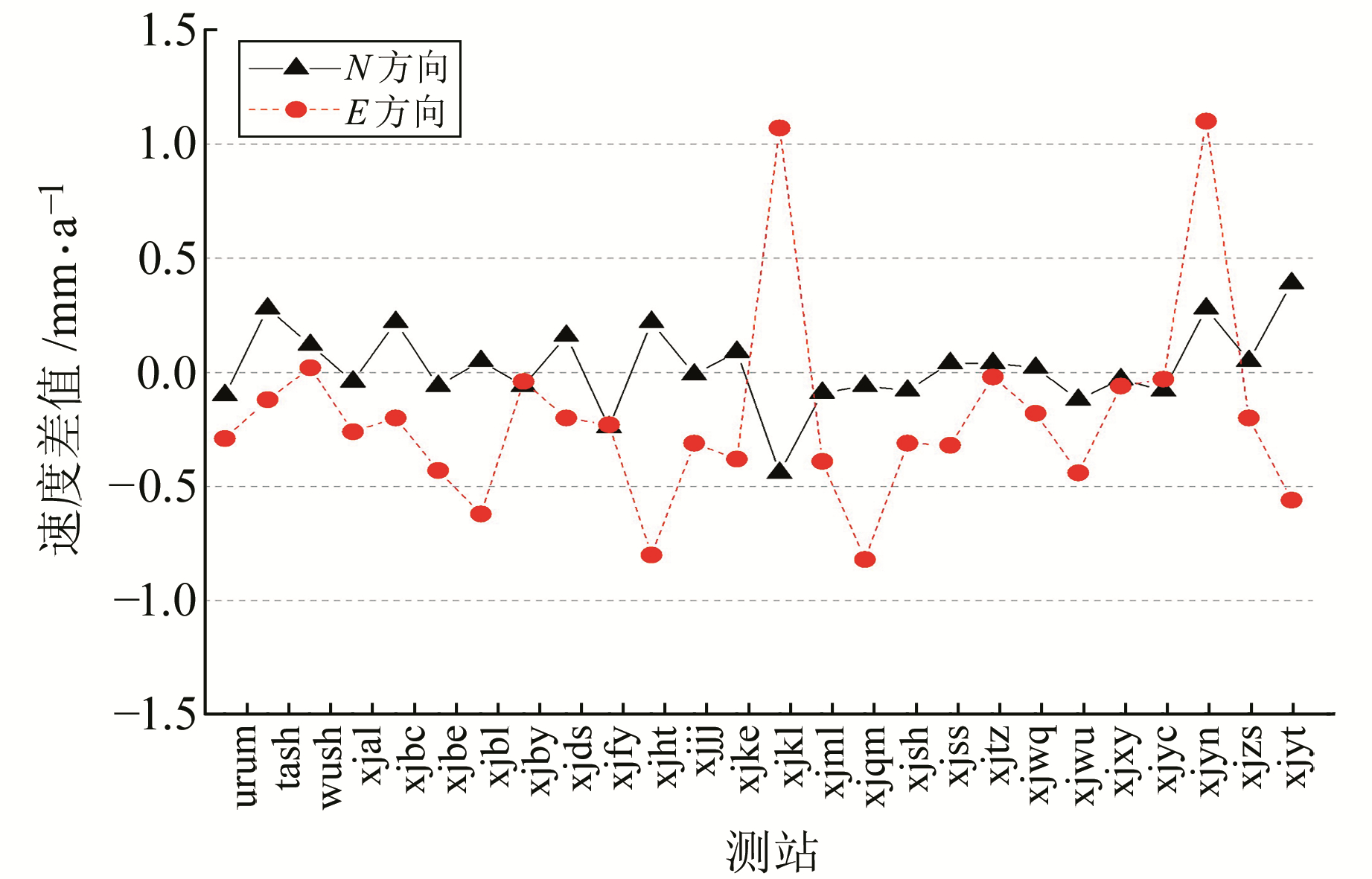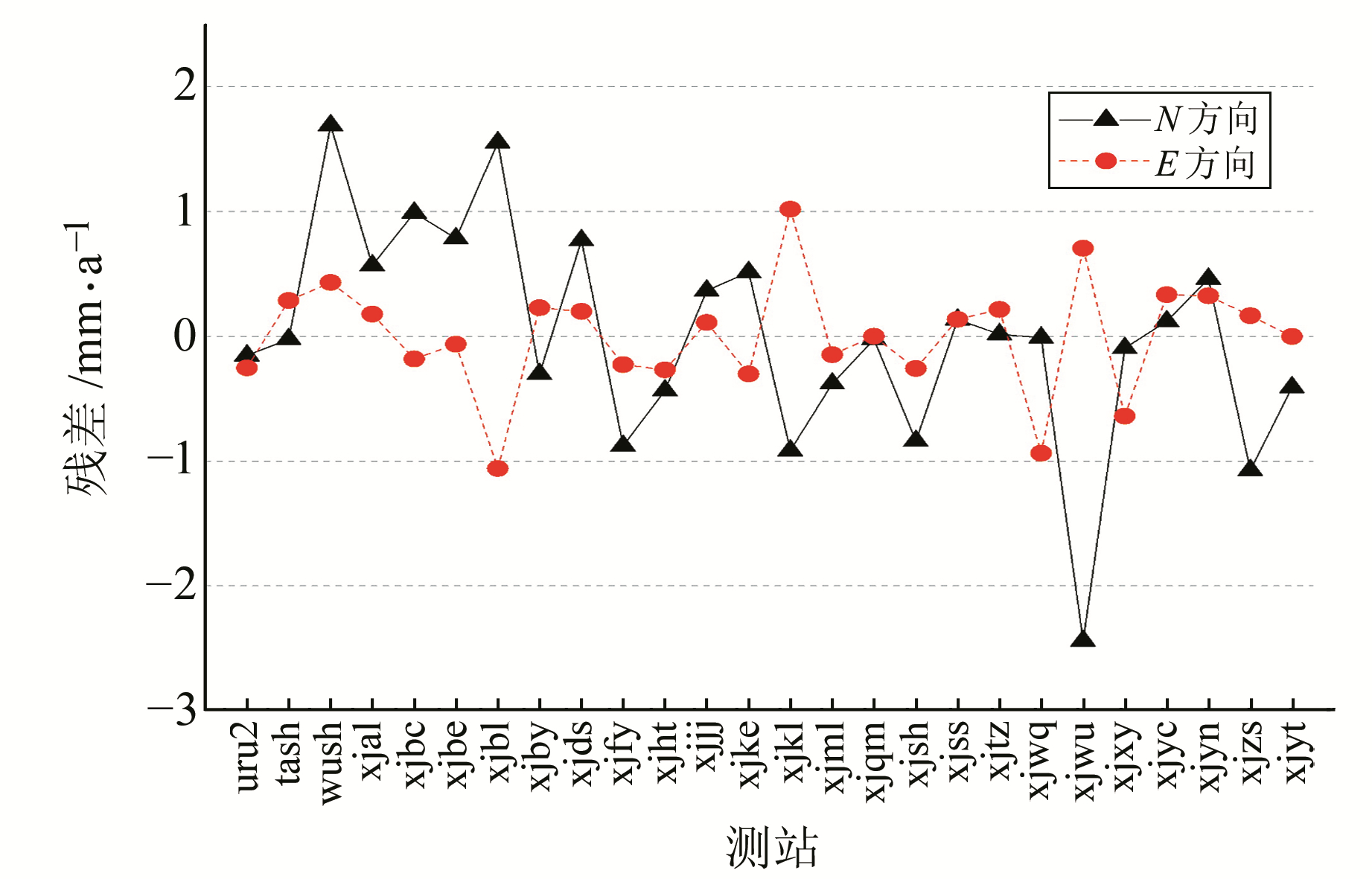﻿ 基于精密单点定位和自适应最小二乘配置建立新疆水平速度场格网模型文章快速检索 高级检索
 大地测量与地球动力学2019, Vol. 39Issue (10): 1058-1062  DOI: 10.14075/j.jgg.2019.10.014### 引用本文GUO Xinwei, TIAN Jie, NIE Jianliang, et al. Establishment of Horizontal Velocity Field Grid Model in Xinjiang Based on Precise Point Positioning and Adaptive Least-Squares Collocation[J]. Journal of Geodesy and Geodynamics, 2019, 39(10): 1058-1062.### Foundation support

National Natural Science Foundation of China, No. 41574003, 41774004, 41474015, 41604001; Special Project of Basic Work of Science and Technology, Ministry of Science and Technology, No. 2015FY210400; Science and Technology Innovation Fund of SHASG; Open Fund of Key Laboratory for Modern Engineering Surveying of NASMG, No. TJES1504; Open Fund of Key Laboratory of Geo-Informatics Engineering, NASMG.

### 第一作者简介

GUO Xinwei, assistant engineer, majors in geodetic data processing，E-mail: xwguo6@gmail.com.

### 文章历史

1. 国家自然资源部大地测量数据处理中心，西安市友谊东路334号，710054;
2. 沈阳市勘察测绘研究院，沈阳市南三好街1号，110004;
3. 新疆维吾尔自治区测绘科学研究院，乌鲁木齐市中山路462号，830002

1 GNSSer软件

GNSSer是一款高精度GNSS解算软件，可进行精密单点定位和双差定位，支持GPS、GLONASS、BDS和Galileo系统的解算，软件基于多核并行和分布式网络技术设计，运算能力强[11-13]。为检验软件精密单点定位精度和计算速度，软件设计者选取全球420个IGS站2013-12-29~2014-01-31的观测数据，以IGS公布的单日解坐标为真值，进行差值统计，主要参数设置见表 1。结果表明，利用GNSSer进行精密单点定位时，非差浮点解水平方向精度优于3 mm，高程方向精度优于6 mm，且多天解精度稳定性较好。此外，多节点多核环境下的非差计算效率得到较大提高。表 1 主要参数设置 Tab. 1 Main parameters setting
2 测站水平方向线性速度计算

 $\begin{array}{c} {N_i} = a\Delta t + b + {A_{\rm{1}}}{\rm{sin}}(2{\rm{ \mathsf{ π} }}\Delta t) + {B_1}\cos (2{\rm{ \mathsf{ π} }}\Delta t) + \\ {A_2}\sin (4{\rm{ \mathsf{ π} }}\Delta t) + {B_2}\cos (4{\rm{ \mathsf{ π} }}\Delta t) \end{array}$ (1)

3 格网速度场建模

 $\mathit{\boldsymbol{L}} = \mathit{\boldsymbol{AX}} + \mathit{\boldsymbol{BS}} + \mathit{\boldsymbol{ \boldsymbol{\varDelta} }}$ (2)

 $\left\{ {\begin{array}{*{20}{l}} {\mathit{\boldsymbol{\hat X}} = {{({\mathit{\boldsymbol{A}}^{\rm{T}}}\mathit{\boldsymbol{ \boldsymbol{\varSigma} }}_L^{ - 1}\mathit{\boldsymbol{A}})}^{{\rm{ - }}1}}{\mathit{\boldsymbol{A}}^{\rm{T}}}\mathit{\boldsymbol{ \boldsymbol{\varSigma} }}_L^{{\rm{ - }}1}\mathit{\boldsymbol{L}}}\\ {\mathit{\boldsymbol{\hat S}} = {\mathit{\boldsymbol{ \boldsymbol{\varSigma} }}_S}{\mathit{\boldsymbol{B}}^{\rm{T}}}\mathit{\boldsymbol{ \boldsymbol{\varSigma} }}_L^{{\rm{ - }}1}(\mathit{\boldsymbol{L}} - \mathit{\boldsymbol{A\hat X}})}\\ {\mathit{\boldsymbol{\hat S'}} = {\mathit{\boldsymbol{ \boldsymbol{\varSigma} }}_{S'S}}\mathit{\boldsymbol{ \boldsymbol{\varSigma} }}_S^{ - 1}\mathit{\boldsymbol{\hat S}}} \end{array}} \right.$ (3)

 $\alpha = \frac{{\hat \sigma _{0S}^{2(i)}}}{{\hat \sigma _{0\Delta }^{2(i)}}}$ (4)

 $\mathit{\boldsymbol{ \boldsymbol{\varSigma} }}_{\Delta} ^{^{(i + 1)}} = \frac{1}{\alpha }\mathit{\boldsymbol{ \boldsymbol{\varSigma} }}_{\Delta} ^{^{(i)}}$ (5)

4 实验及分析 4.1 数据处理及分析图 1 新疆连续运行基准站站点分布 Fig. 1 The distribution of continuously operating reference stations in Xinjiang表 2 GAMIT、GNSSer解算用时统计 Tab. 2 The time spending on solving observation files by GAMIT and GNSSer图 2 GNSSer与GAMIT/GLOBK获得的测站速度在N、E方向的差值 Fig. 2 Station velocity difference obtained by GNSSer and GAMIT/GLOBK in the N and E direction表 3 测站速度差值统计 Tab. 3 Statistics of station velocity difference

4.2 新疆速度场表 4 速度场格网模型精度 Tab. 4 The precision of velocity field grid model图 3 新疆水平方向格网速度场模型 Fig. 3 Horizontal velocity field grid model in Xinjiang图 4 测站拟合残差 Fig. 4 Station fitting residuals

5 结语

  刘经南, 姚宜斌, 施闯, 等. 多面函数拟合法及其在建立中国地壳平面运动速度场模型中的应用研究[J]. 武汉大学学报:信息科学版, 2001, 26(6): 500-503 (Liu Jingnan, Yao Yibin, Shi Chuang, et al. Hardy Function Interpolation and Its Applications to the Establishment of Crustal Movement Speed Field[J]. Geomatics and Information Science of Wuhan University, 2001, 26(6): 500-503) (0)  魏子卿, 刘光明, 吴富梅. 2000中国大地坐标系:中国大陆速度场[J]. 测绘学报, 2011, 40(4): 403-410 (Wei Ziqing, Liu Guangming, Wu Fumei. China Geodetic Coordinate System 2000: Velocity Field in Mainland China[J]. Acta Geodaetica et Cartographica Sinica, 2011, 40(4): 403-410) (0)  任雅奇.基于GPS数据的中国地壳运动速度场模型的建立[D].郑州: 信息工程大学, 2012 (Ren Yaqi. Establishment of Chinese Crustal Movement Velocity Model Based on the GPS Data[D]. Zhengzhou: Information Engineering University, 2012) http://cdmd.cnki.com.cn/Article/CDMD-90005-1013161053.htm (0)  刘经南, 姚宜斌, 施闯. 中国地壳运动整体速度场模型的建立方法研究[J]. 武汉大学学报:信息科学版, 2002, 27(4): 331-336 (Liu Jingnan, Yao Yibin, Shi Chuang. Method for Establishing the Speed Field Model of Crustal Movement in China[J]. Geomatics and Information Science of Wuhan University, 2002, 27(4): 331-336) (0)  曾安敏, 秦显平, 刘光明, 等. 中国大陆水平运动速度场的多面函数模型[J]. 武汉大学学报:信息科学版, 2013, 38(4): 394-398 (Zeng Anmin, Qin Xianping, Liu Guangming, et al. Hardy Multi-Quadric Fitting Model of Chinese Mainland Horizontal Crustal Movement[J]. Geomatics and Information Science of Wuhan University, 2013, 38(4): 394-398) (0)  于亮, 朱璇, 陈永祥, 等. 基于CMONOC建立和评估中国大陆地壳运动速度场模型[J]. 大地测量与地球动力学, 2017, 37(7): 704-708 (Yu Liang, Zhu Xuan, Chen Yongxiang, et al. The Building and Estimating of Velocity Field in Mainland China Based on CMONOC[J]. Journal of Geodesy and Geodynamics, 2017, 37(7): 704-708) (0)  柴洪洲, 崔岳, 明锋. 最小二乘配置方法确定中国大陆主要块体运动模型[J]. 测绘学报, 2009, 38(1): 61-65 (Chai Hongzhou, Cui Yue, Ming Feng. The Determination of Chinese Mainland Crustal Movement Model Using Least-Squares Collocation[J]. Acta Geodaetica et Cartographica Sinica, 2009, 38(1): 61-65 DOI:10.3321/j.issn:1001-1595.2009.01.011) (0)  江在森, 刘经南. 应用最小二乘配置建立地壳运动速度场与应变场的方法[J]. 地球物理学报, 2010, 53(5): 1 109-1 117 (Jiang Zaisen, Liu Jingnan. The Method in Establishing Strain Field and Velocity Field of Crustal Movement Using Least Squares Collocation[J]. Chinese Journal of Geophysics, 2010, 53(5): 1 109-1 117) (0)  张菊清, 张亮. 基于Helmert方差分量估计的拟合推估法及其在地图数字化误差纠正中的应用[J]. 测绘通报, 2008(2): 35-37 (Zhang Juqing, Zhang Liang. The Collocation Method Based on Helmert Variance Component Estimation and Its Application in Errors Correction of Digital Maps[J]. Bulletin of Surveying and Mapping, 2008(2): 35-37) (0)  程义军, 程广义, 刘威. 精密单点定位在GNSS区域网监测中的应用[J]. 大地测量与地球动力学, 2015, 35(1): 97-99 (Cheng Yijun, Cheng Guangyi, Liu Wei. Application of Precise Point Positioning on GNSS Regional Network Monitoring[J]. Journal of Geodesy and Geodynamics, 2015, 35(1): 97-99) (0)  崔阳, 吕志平, 张友阳, 等. 一种GNSS大网数据快速高效处理策略[J]. 大地测量与地球动力学, 2015, 35(3): 383-386 (Cui Yang, Lü Zhiping, Zhang Youyang, et al. Study of Cycle-Slip Detection Using BDS Triple-Frequency Geometry-Free and Ionosphere-Free Combination[J]. Journal of Geodesy and Geodynamics, 2015, 35(3): 383-386) (0)  陈正生, 吕志平, 崔阳, 等. 大规模GNSS数据的分布式处理与实现[J]. 武汉大学学报:信息科学版, 2015, 40(3): 384-389 (Chen Zhengsheng, Lü Zhiping, Cui Yang, et al. Implementation of Distributed Computing with Large-Scale GNSS Data[J]. Geomatics and Information Science of Wuhan University, 2015, 40(3): 384-389) (0)  李林阳.大规模GNSS网云计算方法与实践[D].郑州: 信息工程大学, 2016 (Li Linyang. Cloud Computing Method and Practice of Large Scale GNSS Network[D].Zhengzhou: Information Engineering University, 2016) (0)  杨元喜. 抗差估计理论及其应用[M]. 北京: 八一出版社, 1993 (Yang Yuanxi. Robust Estimation and Its Applications in Geodesy[M]. Beijing: Bayi Publishing House, 1993) (0)  Dong D, Fang P, Bock Y, et al. Spatiotemporal Filtering Using Principal Component Analysis and Karhunen-Loeve Expansion Approaches for Regional GPS Network Analysis[J]. Journal of Geophysical Research: Solid Earth, 2006, 111(B3) (0)  Wdowinski S, Bock Y, Zhang J, et al. Southern California Permanent GPS Geodetic Array: Spatial Filtering of Daily Positions for Estimating Coseismic and Postseismic Displacements Induced by the 1992 Landers Earthquake[J]. Journal of Geophysical Research: Solid Earth, 1997, 102(B8): 18 057-18 070 DOI:10.1029/97JB01378 (0)
Establishment of Horizontal Velocity Field Grid Model in Xinjiang Based on Precise Point Positioning and Adaptive Least-Squares Collocation
GUO Xinwei1TIAN Jie1     NIE Jianliang1     TAI He2     JIN Xinyang1     DENG He3     ZHANG Tao1
1. Geodetic Data Processing Centre, MNR, 334 East-Youyi Road, Xi'an 710054, China;
2. Shenyang Geotechnical Investigation and Research Institute, 1 South-Sanhao Street, Shenyang 110004, China;
3. Academy of Surveying and Mapping of Xinjiang Uygur Autonomous Region, 462 Zhongshan Road, Urumqi 830002, China
Abstract: In order to solve the inefficient problem in calculating station coordinates with double-difference positioning, GNSSer PPP mode is introduced to speed up the solution process by multicore parallel operation. The precision of velocity field model is reduced due to the random signal contained in the station velocity, so least-squares collocation is used to estimate the random signal, and the Helmert estimation of variance components is introduced to adjust the unreasonable relationship between the noise covariance matrix and the signal covariance matrix. Then, a more accurate velocity field model can be established. Taking the continuously operating reference stations of the CMONOC in Xinjiang from 2011 to 2017 as an example, GNSSer PPP mode is used to obtain the coordinate time series to improve the efficiency of the calculation. The reliability and efficiency of GNSSer are verified. It is also confirmed that the PPP can obtain the velocity information, which is substantially consistent with the double-difference positioning, the difference is within 1.5 mm/a. The established horizontal velocity field grid model in Xinjiang shows that the movement velocity in Xinjiang is 27.1-34.8 mm/a, and the overall trend is north to east. From southwest to northeast in Xinjiang, the direction of motion is deflected from north to east.
Key words: GNSSer; precise point positioning(PPP); velocity field; Helmert estimation of variance components; Xinjiang